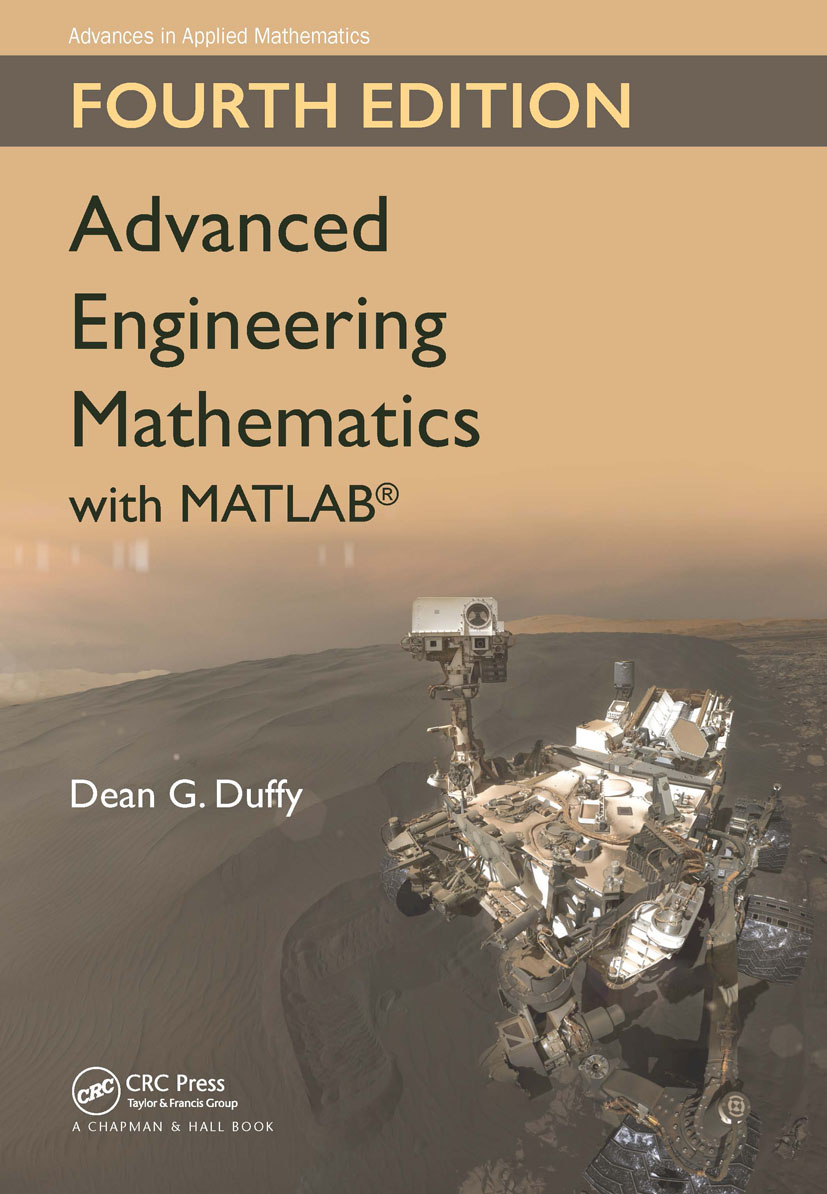Advanced Engineering Mathematics with MATLAB

4th Edition

Chapman and Hall/CRC

980 pages | 104 Color Illus. | 239 B/W Illus.

Purchasing Options:\$ = USD
Hardback: 9781498739641
pub: 2016-12-22
SAVE ~\$29.00
\$145.00
\$116.00
x
eBook (VitalSource) : 9781315369280
pub: 2016-12-12
from \$72.50

FREE Standard Shipping!

Description

Advanced Engineering Mathematics with MATLAB, Fourth Edition builds upon three successful previous editions. It is written for today’s STEM (science, technology, engineering, and mathematics) student.

Three assumptions under lie its structure: (1) All students need a firm grasp of the traditional disciplines of ordinary and partial differential equations, vector calculus and linear algebra. (2) The modern student must have a strong foundation in transform methods because they provide the mathematical basis for electrical and communication studies. (3) The biological revolution requires an understanding of stochastic (random) processes. The chapter on Complex Variables, positioned as the first chapter in previous editions, is now moved to Chapter 10.

The author employs MATLAB to reinforce concepts and solve problems that require heavy computation. Along with several updates and changes from the third edition, the text continues to evolve to meet the needs of today’s instructors and students.

Features:

• Complex Variables, formerly Chapter 1, is now Chapter 10.
• A new Chapter 18: Itô’s Stochastic Calculus.
• Implements numerical methods using MATLAB, updated and expanded
• Takes into account the increasing use of probabilistic methods in engineering and the physical sciences
• Includes many updated examples, exercises, and projects drawn from the scientific and engineering literature
• Draws on the author’s many years of experience as a practitioner and instructor
• Gives answers to odd-numbered problems in the back of the book
• Offers downloadable MATLAB code at www.crcpress.com

CLASSIC ENGINEERING MATHEMATICS

First-Order Ordinary Differential Equations

Classification of Differential Equations

Separation of Variables

Homogeneous Equations

Exact Equations

Linear Equations

Graphical Solutions

Numerical Methods

Higher-Order Ordinary Differential Equations

Homogeneous Linear Equations with Constant Coefficients

Simple Harmonic Motion

Damped Harmonic Motion

Method of Undetermined Coefficients

Forced Harmonic Motion

Variation of Parameters

Euler-Cauchy Equation

Phase Diagrams

Numerical Methods

Linear Algebra

Fundamentals of Linear Algebra

Determinants

Cramer’s Rule

Row Echelon Form and Gaussian Elimination

Eigenvalues and Eigenvectors

Systems of Linear Differential Equations

Matrix Exponential

Vector Calculus

Review

Divergence and Curl

Line Integrals

The Potential Function

Surface Integrals

Green’s Lemma

Stokes’ Theorem

Divergence Theorem

Fourier Series

Fourier Series

Properties of Fourier Series

Half-Range Expansions

Fourier Series with Phase Angles

Complex Fourier Series

The Use of Fourier Series in the Solution of Ordinary Differential Equations

Finite Fourier Series

The Sturm-Liouville Problem

Eigenvalues and Eigenfunctions

Orthogonality of Eigenfunctions

Expansion in Series of Eigenfunctions

A Singular Sturm-Liouville Problem: Legendre’s Equation

Another Singular Sturm-Liouville Problem: Bessel’s Equation

Finite Element Method

The Wave Equation

The Vibrating String

Initial Conditions: Cauchy Problem

Separation of Variables

D’Alembert’s Formula

Numerical Solution of the Wave Equation

The Heat Equation

Derivation of the Heat Equation

Initial and Boundary Conditions

Separation of Variables

Numerical Solution of the Heat Equation

Laplace’s Equation

Derivation of Laplace’s Equation

Boundary Conditions

Separation of Variables

Poisson’s Equation on a Rectangle

Numerical Solution of Laplace’s Equation

Finite Element Solution of Laplace’s Equation

TRANSFORM METHODS

Complex Variables

Complex Numbers

Finding Roots

The Derivative in the Complex Plane: The Cauchy-Riemann Equations

Line Integrals

The Cauchy-Goursat Theorem

Cauchy’s Integral Formula

Taylor and Laurent Expansions and Singularities

Theory of Residues

Evaluation of Real Definite Integrals

Cauchy’s Principal Value Integral

Conformal Mapping

The Fourier Transform

Fourier Transforms

Fourier Transforms Containing the Delta Function

Properties of Fourier Transforms

Inversion of Fourier Transforms

Convolution

Solution of Ordinary Differential Equations

The Solution of Laplace’s Equation on the Upper Half-Plane

The Solution of the Heat Equation

The Laplace Transform

Definition and Elementary Properties

The Heaviside Step and Dirac Delta Functions

Some Useful Theorems

The Laplace Transform of a Periodic Function

Inversion by Partial Fractions: Heaviside’s Expansion Theorem

Convolution

Integral Equations

Solution of Linear Differential Equations with Constant Coefficients

Inversion by Contour Integration

The Solution of the Wave Equation

The Solution of the Heat Equation

The Superposition Integral and the Heat Equation

The Solution of Laplace’s Equation

The Z-Transform

The Relationship of the Z-Transform to the Laplace Transform

Some Useful Properties

Inverse Z-Transforms

Solution of Difference Equations

Stability of Discrete-Time Systems

The Hilbert Transform

Definition

Some Useful Properties

Analytic Signals

Causality: The Kramers-Kronig Relationship

Green’s Functions

What Is a Green’s Function?

Ordinary Differential Equations

Joint Transform Method

Wave Equation

Heat Equation

Helmholtz’s Equation

Galerkin Methods

STOCHASTIC PROCESSES

Probability

Review of Set Theory

Classic Probability

Discrete Random Variables

Continuous Random Variables

Mean and Variance

Some Commonly Used Distributions

Joint Distributions

Random Processes

Fundamental Concepts

Power Spectrum

Two-State Markov Chains

Birth and Death Processes

Poisson Processes

Itˆo’s Stochastic Calculus

Random Differential Equations

Random Walk and Brownian Motion

Itˆo’s Stochastic Integral

Itˆo’s Lemma

Stochastic Differential Equations

Numerical Solution of Stochastic Differential Equations

Dean G. Duffy is a former mathematics instructor at the US Naval Academy and US Military Academy. He spent 25 years working on numerical weather prediction, oceanic wave modeling, and dynamical meteorology at NASA’s Goddard Space Flight Center. Prior to this, he was a numerical weather prediction officer in the US Air Force. He earned his Ph.D. in meteorology from MIT. Dr. Duffy has written several books on transform methods, engineering mathematics, Green’s functions, and mixed boundary value problems.